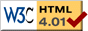# Reaction Force Pendulum

previous next
• Sim
• Graph
• Time Graph
• Multi Graph

Simulation of a pendulum using reaction forces (on left in red), along with a comparison simulation using the equations of motion (on right in blue).

You can change parameters in the simulation such as gravity, starting angle, length of the rod, radius of the disk, mass, and separation between the two pendulums.

This contrasts two very different ways of solving the same simulation:

1. Find the equations of motion using Newton's equations of motion. The blue pendulum on the right does this, it is the same simulation as the ideal pendulum; see that page for the math.
2. Calculate the reaction forces needed at each moment to maintain the pivot point at the same location. The red pendulum on the left does this. The reaction forces are visible as the dashed green lines.

The "classic" blue pendulum is the theoretically correct solution. So it is a good check that the reaction force pendulum is working correctly.

The pendulum is regarded as a rigid body consisting of a uniform disk at end of a massless rigid arm. We find the reaction forces by solving a matrix equation, following the steps shown on the rigid body contact forces page.

The math used here is the same as that used by the 2D Rigid Body Physics Engine. But this simulation is "hard-coded" for this particular setup. In contrast, the physics engine is far more capable: it is designed to work on any group of rigid bodies, connected by joints, springs, ropes, or rods, handling any forces applied to the bodies, as well as collisions.

The point of this simulation is to show a simpler example of the math involved in finding contact forces. This is an example of finding contact forces without writing a highly general (and complex) physics engine.

To match the motion of the two simulations, the length of the rods are slightly different. This is necessary because the reaction force pendulum models the pendulum as a rigid disc with mass distributed evenly, whereas the classic ideal pendulum models the pendulum as a point mass at the end of a massless rod. See the ReactionPendulumApp documentation for how the equivalent lengths are calculated.

Also available are: open source code, documentation and a simple-compiled version which is more customizable.

This web page was first published November 2016.

previous next